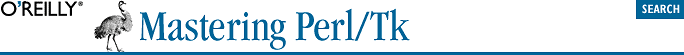home | O'Reilly's CD bookshelfs | FreeBSD | Linux | Cisco | Cisco Exam## 9.4. Using bind with a Canvas

```\$canvas = \$mw->Canvas( );
\$canvas->CanvasBind("<Button-1>", sub { print "bind!\n"; });```
```\$canvas = \$mw->Scrolled("Canvas");
\$real_canvas = \$canvas->Subwidget("canvas");
\$real_canvas->CanvasBind("<Button-1>", sub { print "bind!\n" });```

Other than this one small annoyance, bind works just as you would expect. This example prints the Canvas coordinate you clicked on:

```\$c = \$mw->Scrolled("Canvas")->pack( );
\$canvas = \$c->Subwidget("canvas");
\$canvas->CanvasBind("<Button-1>", [ \&print_xy, Ev('x'), Ev('y') ]);
sub print_xy {
my (\$canv, \$x, \$y) = @_;
print "(x,y) = ", \$canv->canvasx(\$x), ", ", \$canv->canvasy(\$y), "\n";
}```

To summarize, use bind to create event bindings to Canvas items, and use CanvasBind to create event bindings to the Canvas widget.